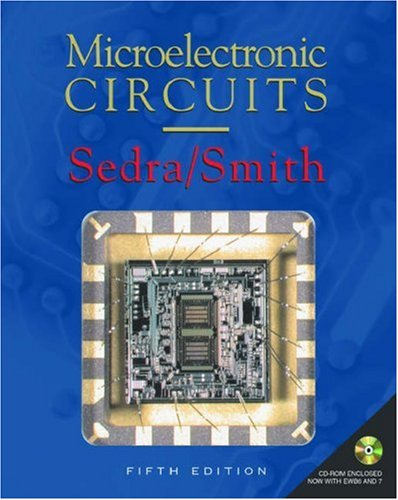## Sunday, September 09, 2012

### Introduction to Integrated Circuits PDF SLIDESIntroduction to Integrated Circuits

Instructor: Prof . King
Textbook: micro electronic circuit
Download Slides from here

Introduction
Lecture 1: Course overview and introduction; analog vs. digital signals

Circuit Analysis
Lecture 2: Overview of circuit analysis, electrical quantities, ideal basic circuit element, sign conventions
Lecture 3: Power calculations; circuit elements (voltage and current sources, resistor); Kirchhoff's laws
Lecture 4: Circuit element I-V characteristics; construction of a circuit model; Kirchhoff's laws -- a closer look
Lecture 5: Resistors in series; voltage divider; resistors in parallel; current divider; measuring current and voltage
Lecture 6: Wheatstone bridge circuit; delta-to-wye equivalent circuits; node-voltage analysis method
Lecture 7: Node-voltage analysis of circuit with dependent sources; source transformations; mesh-current analysis method
Lecture 8: Thevenin and Norton equivalent circuits; maximum power transfer; superposition

The Operational Amplifier
Lecture 9: Op amp terminals and voltages; feedback; comparator circuits; ideal op amp; unity-gain voltage follower circuit
Lecture 10: Inverting-amplifier circuit; summing-amplifer circuit; non-inverting amplifier circuit; difference-amplifier circuit
Lecture 11: Op amp circuit analysis; the capacitor

First-Order Circuits
Lecture 12: Capacitors in series and in parallel; the inductor; inductors in series and in parallel
Lecture 13: Mutual inductance; natural response of an RL circuit
Lecture 14: Natural response of RC circuit
Lecture 15: General transient response of RL and RC circuits; application to digital logic gate
Lecture 16: Propagation delay; energy consumption of simple RC circuit

Semiconductor Devices and Technology
Lecture 17: Semiconductor materials; properties of Si; doping
Lecture 18: Generation and recombination; charge-carrier transport; resistivity
Lecture 19: IC resistors; the p-n junction diode (depletion region, built-in voltage, I-V characteristic, capacitance)
Lecture 20: Reverse breakdown; load-line analysis method; diode models; diode applications
Lecture 21: More diode applications; introduction to the MOSFET
Lecture 22: The MOSFET as a controlled resistor; current saturation; channel-length modulation; velocity saturation
Lecture 23: MOSFET ID-VGS characteristic; MOSFET circuit models

Transistor Amplifier Circuits
Lecture 24: The common-source amplifier
Lecture 25: General transconductance amplifier; summary of MOSFET

Digital Integrated Circuits
Lecture 26: Logic functions; NMOS logic gates; noise margin; the CMOS inverter
Lecture 27: Current flow in CMOS inverter during switching; CMOS logic gates; the body effect
Lecture 28: Review (various examples relevant for Midterm #2)
Lecture 29: Logic circuit synthesis; minimization of logic circuits
Lecture 30: Sequential logic circuits
Lecture 31: Fan-out; propagation delay; CMOS power consumption; timing diagrams
Lecture 32: Computing the output capacitance; history of IC devices and technology
Lecture 33: Basic IC fabrication technology
Lecture 34: Modern IC fabrication technology
Lecture 35: Device isolation methods; electrical contacts to Si; mask layout conventions; process flow examples
Lecture 36: CMOS process flow and layout; circuit extraction from layout
Lecture 37: Interconnect parameters; interconnect modeling
Lecture 38: Propagation delay with interconnect; inter-wire capacitance; pi model for capacitive coupling; capacitive coupling effects
Lecture 39: Transistor scaling; interconnect scaling
Lecture 40: Microelectronics technology in the 21st century
Lecture 41: Example problems for the final exam